Special Offer - Enroll Now and Get 2 Course at ₹25000/- Only Explore Now!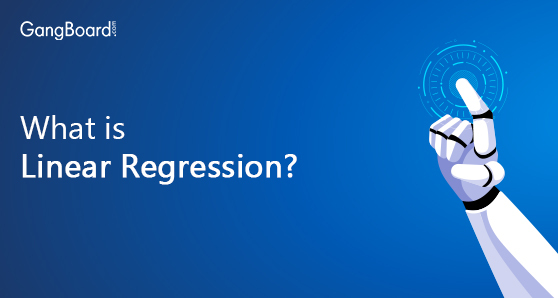# Linear Regression

May 24th, 2019

## What is Linear Regression?

We all understand and appreciate the powers of a Computer. It can solve the most complex of problems with a certain ease that humans are not minutely capable of. A simple example is – 23654 multiplied by 78593. A computer solves it by the time we read it the second time.
All this greatness of the computer’s capabilities has a prerequisite, though. The instructions are to be mentioned and a computer would religiously follow each of the steps and provides us with the solution. Providing these instructions to a computer is also called writing a variable program on a computer to deliver a certain task (add or multiply or copy or paste, etc.). You need to provide the inputs and the steps to follow. Then the output is produced.
Imagine a world where all we need to do is give information as inputs and we get the solution as an output where we don’t need to explicitly write programs for everything that the computer has to do. That’s what Machine Learning does.

### Machine Learning

Machine Learning is essentially a study of algorithms that computers can use to perform tasks without actually providing any explicit instructions. The insights would be generated more through the available patterns in the data and the potential inferences.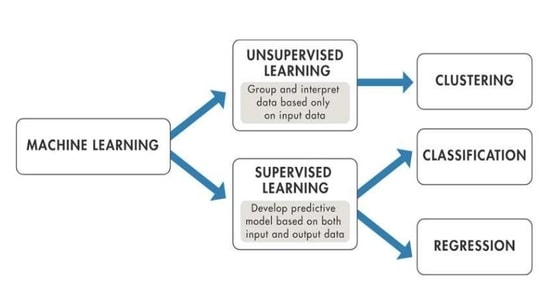A few Machine learning applications from our everyday lives –

#### Virtual Personal Assistants:

Google Assistant or Siri are applications that take your language as an input, understand the need from the sentences and deliver the results. Want to try, pick up your phone and say – “ OK Google, what’s the temperature today?” Or “Hey Siri, how far is Delhi from my place?”. You’ll see magic.

#### Traffic Predictions:

Have you ever set a specific destination on Google Maps and looked at its prediction of the amount of time it would take you to reach there? That’s machine learning. And, the time predictions are eerily close to the realities too, a mark that the computers are learning well.

#### Social Media Services:

Have you observed the friend recommendations that Facebook or Instagram throw at us to further follow? That analysis of “People you may know” is a machine learning exercise.

### Learning Ways

Let’s put the computers aside for a bit. As humans surviving in society, we face a variety of problems in front of us and we have come up with a range of techniques to solve these.
One of the problems can be to see by how much your bank balance might be affected if you eat pizza every day.
Another is to understand if spending time in the morning playing cricket would mean if you can catch the bus or not to the college.
Or, What kind of friend would you like to have in your life if you have to pick from a group of strangers?
The questions differ on various levels. Now, back to the computers. If we want computers to learn how to solve these problems, we need to start separating problems into different categories. Broadly –

#### Supervised Learning:

Supervised learning is one amongst all the techniques of Machine Learning. As you know we opt supervised learning when you know what you want the model (algorithm) to do. In short, supervised learning can be used when we know the target of the model being built.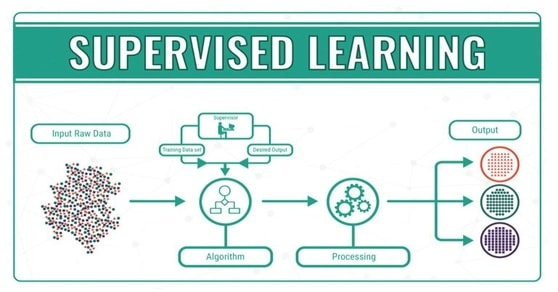In the examples cited above, you know that the number of pizzas you eat would have an effect on the bank balance. You also know that depending on how far the destination you’ve to reach, the time you’d have to take to reach there would depend on. There’s a pattern here. You know what inputs (pizzas you eat, the distance you have to travel) would affect the potential outcomes (your bank balance and the time of travel). So, this learning involves a certain relation that can map inputs to an output based on a lot of such input-output pairs.

#### Unsupervised Learning:

Imagine you’ve visited your cousin’s place far away and he took you to a party of total strangers, with no information about anyone around. How would you go about this situation and try to mingle? You’ll identify a few people to be drinking, then a few others to be eating, a few watching sports, etc. You identify patterns of what’s happening around you to blend better. This is a decent example of Unsupervised learning. In this case, the computer systems would only be provided with inputs and are asked to find patterns and associations among the data. These insights can further be used to come up with interesting outcomes, like – “You may know them” recommendations from the Social Media, people who buy x would also buy y in e-commerce, etc.

### Supervised Learning

Let’s limit ourselves to supervised learning this time around. Broadly, this kind of learning can be divided into two categories .
There are two types of Supervised learning:

• Classification
• Regression

Basically Regression and classification are called the two problems of supervised learning. These define two important features of Machine learning.
Which are:

• Continuous Feature
• Categorical Feature

#### Regression:

Regression defines the continuous feature of supervised learning. Continuous feature can be used when the elements of the algorithm/problem share a mathematical relation between them. It is used when you don’t know all the possible outcomes of the model you are building. Here the possible outcome can be any numerical value with a particular continuous range. Example: Linear Regression, Logistic Regression, etc.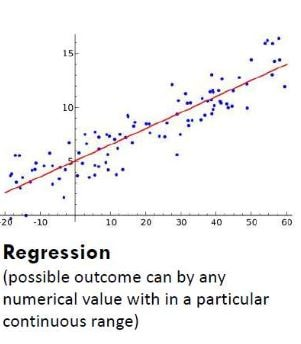When a continuous change in inputs leads to a continuous change in the output, then it is a regression problem. The cost of the flat increases basis the size of it. The bank balance decreases on the basis of the number of pizzas eaten.

#### Classification:

Classification is nothing but the categorical feature of supervised learning. Categorical feature is used when there is no logic involved in the problem or when the parameters involved share no mathematical relation between them. Classification is used If you know all the possible answers that algorithm is going to predict.
Most possible outcomes here are binary (0, 1). Example: Decision tree, Random forest, etc.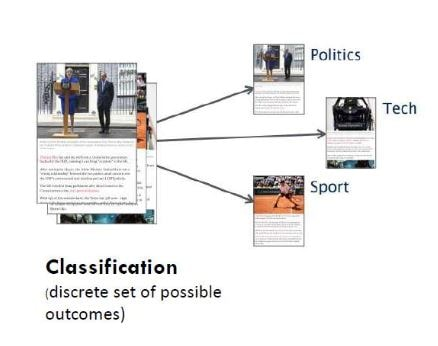If for a wide range of inputs, there are only a few possible outputs possible, it is a classification problem. Is the mail you’ve received spam or regular mail. Does the tumor with a certain size is malignant or not.
Linear Regression is one of the most basic and simplest of algorithms that are used to solve regression problems.

### What is Linear Regression?

To put it in layman terms, when we try to find the best fit linear curve between the inputs and outputs, it is a linear regression exercise. For example, the number of days one would eat pizzas can potentially have a linear relation with the decreasing bank balance. So, we can imagine this exercise as the one where we try to identify the line that would best fit the available input and output metrics. Upon finding it, we would further use this line to predict the potential outputs for a given input.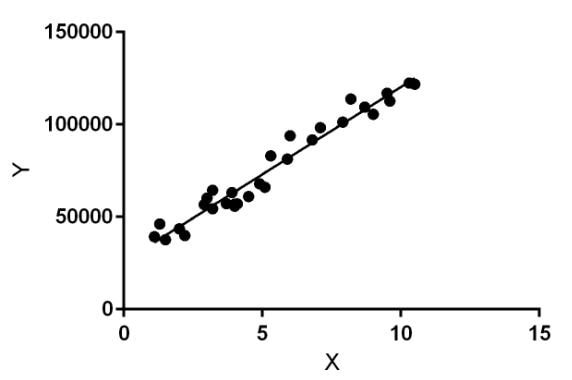To further appreciate the intricacies of the Linear regression, let us dwell a little into the elements that are involved in it:

### Features & Output:

a feature is an individual property or characteristic, that is measurable, of a phenomenon being observed. In the above-mentioned pizza to bank balance example, the number of pizzas consumed is a metric that would potentially affect the outcome, the bank balance. So, that would become a feature.
Now, out there in the real world, not every form of information might be applicable and relevant in affecting the output. For example, the number hours one would sleep would not have a direct relation to the bank balance and hence would be a bad choice as a feature in this exercise.
It is important to choose the relevant features that can potentially affect the output while working on the regression model. Else, the accuracy and the prediction capacity of the model go down.
The entire data set of inputs mapped to outputs is generally divided into two categories – Training data and Testing Data, the former used to train the algorithm to get the prediction algorithm right and the latter used to test the accuracy of the landed prediction algorithm.

#### Hypothesis:

As mentioned, linear regression is essentially a method of predicting the closest linear algorithm that would fit the data. So, the hypothesis of this model is a linear function with the output related to the features along with the weights (parameters) affecting them. Imagine hypothesis as the prediction relation that we would like to build between inputs and the outputs.
Of course, when we start off, our hypothesis would not be accurate. We don’t know the weights of the inputs (also called parameters) that would predict the most accurate outcome. So, we start with some dummy parameters assigned to start with and then work towards identifying the more accurate set of parameters.
To summarise, Linear Regression is a process in which we try to identify the best fit hypotheses (parameter set) of the infinitely many available for a given set of outputs and inputs. How do we do this?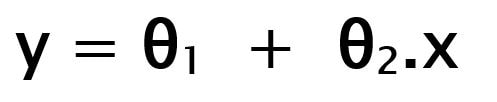Y – Output
Thetas – parameters (weights)
x – features or inputs

#### Cost Function:

With the hypothesis being the predictor function that relates the inputs with the outputs, we would like to know how accurate our relation function is at any given point of time to that of the reality. So, we would like to test it out by comparing the predicted outcomes to the actual outcomes.

##### How do we compare?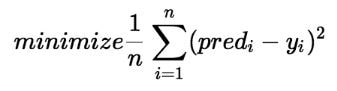One of the robust and efficient ways we use quite often is the root mean square of the difference in the predicted outcomes to the actual outcomes. While predicting, you can either be more than the actual outcome, or lesser. So, just subtracting these values wouldn’t help in understanding the overall prediction capacity. So, by squaring the difference in outcomes, the direction is nullified (squaring would give a positive number even if it’s lesser or higher than the actual value). And, all these squared values generated for all the training examples are further added and called Cost.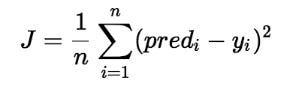The goal is to minimize this cost function – Lesser the cost, more accurate is the model to the training set.

Now, to quickly summarise, we start with a random hypothesis that is a linear curve. Then we identify the cost incurred through this hypothesis by comparing and applying root mean square function on the predicted and actual outcomes. How do we identify the hypothesis that has the lowest value of cost? Enter, gradient descent.
Please note that in this entire exercise of linear regression, the variables that can consistently change are the parameters (weights) attached to the features. So, inherently, the cost function is a function of these parameters alone. So, for a given parameter set values, it would lead to certain cost value.
Now, there would be a certain cost curve evolving in the n-dimensional space (n being one more than the features available). This curve could take any shape but it would be fair to say that it would have its own set of local/global minima and maxima. Gradient Descent is the technique that helps in identifying the parameter set that would lead to the potential minima of the cost function.

#### Learning Rate:

This is the trick. We start with any random parameter values available out there. Then we would calculate the cost function. Now, we would slightly change the values of each of these parameters and calculate the cost function again. If the latest cost value is lesser than the former, we keep making the changes to the parameters until we see that the cost function is not changing anymore. That would mean that we have reached a certain parameter set that would lead to a minimum in the cost function. We would further use this parameter set in our hypothesis and make predictions on the test data and check for accuracy.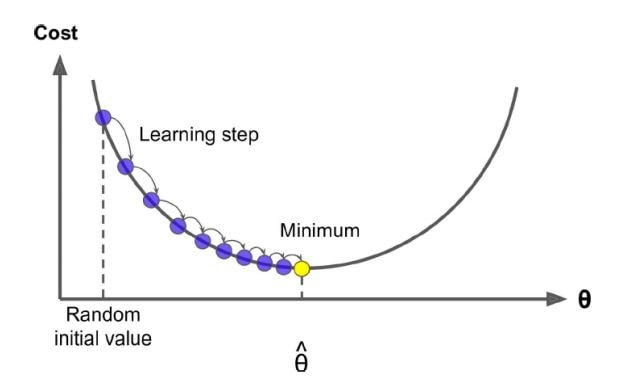One of the important properties in the linear regression exercise is by how much would we like to change the parameters while adjusting for the lowest cost value. Change it too slow, it might take ages to reach the minima and change it by a large margin and we might end up missing the minima. This property that guides the step size of the change in the gradient descent is called learning rate and it is to be tested out as to what learning rate would lead to the most accurate model.

#### Conclusion

Machine learning has gone up on many notches over the last few years. But, the understanding of the Linear Regression model functions as a platform to understand the depths of the Machine Learning algorithms that are available these days.